Cyclist vs boat

The cyclist wants to ride a short distance by boat, but when he stops at the pier, the boat does not pick up passengers yet and is preparing to leave. The cyclist decides that the boat will catch up at the next stop. The stop is 12km far along the water and 17 km along the cycle path. Both the boat and the cyclist will drive towards the next stop at the same time. Calculate the speed at which the cyclist must go to catch the boat at the next stop. Boat floats at a speed of 5 km/h.

Result

v =  7.083 km/h

Solution:

17 = v * (12/5)

12v = 85

v = 8512 ≈ 7.083333

Calculated by our simple equation calculator.

Leave us a comment of this math problem and its solution (i.e. if it is still somewhat unclear...):Be the first to comment!To solve this verbal math problem are needed these knowledge from mathematics:

Do you have a linear equation or system of equations and looking for its solution? Or do you have quadratic equation? Do you want to convert length units?

Next similar math problems:

1. Pedestrian and the cyclist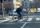Pedestrian and cyclist went against each from the two cities that are distant 40 km. Pedestrian started 30 minutes before the cyclist and walk an average speed of 5 km/h. What speed went cyclist when met after 120 minutes from the start of the pedestrian?
2. Klara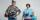Klara and Jitka went on a hiking trip at 13 o'clock at speed 5km/h. At 14 o'clock, Tomas ride on the bike at an average speed of 28 km/h. How many hours and at what distance from the beginning of the road Tomáš caught the two girls?
3. Motorcyclist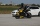From Trutnov, the motorcyclist started at an average speed of 60km/hour. At 12.30hrs the passenger car was started at a speed of 80km/hour. How many hours and at what distance from Trutnov will car catch a motorcycle?
4. Train expres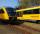At 8.00 strarted from Bratislava to Poprad express Tatry. Cities are distant 340 kilometers. At the same time started from Poprad express Danube to Bratislava. Express Tatry's average speed is 80 km/h, Danube 90 km/h. At what distance from Poprad trains w
5. Sisters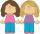Sisters Hanka and Vera goes mostly tograndmother by bicycle. Journey took Hanka 30 minutes and Vera 20 minutes. How long will Vera catch up with Hanka when started from home five minutes later than Hanka?
6. Six yearsIn six years Jan will be twice as old as he was six years ago. How old is he?
7. Simplify 2Simplify expression: 5ab-7+3ba-9
8. GivenGiven 2x =0.125 find the value of x
9. Simple equationSolve for x: 3(x + 2) = x - 18
10. EquationSolve the equation: 1/2-2/8 = 1/10; Write the result as a decimal number.
11. Cars 6At 9:00 am two cars started from the same town and traveled at a rate of 35 miles per hour and the other car traveled at a rate of 40 miles per hour. After how many hours will the cars be 30 miles apart?
12. Greg and BillGreg is 18 years old. He is 6 less than 4 times Bill's age. How old is Bill?
13. Cenda and Pepa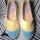Cenda and Pepa went to the event. Cenda started alone. Canda started after him for 20 minutes. How long took Cenda to catch him? Cenda traveling at 15 km/h, and Pepa traveling at 25 km/h.
14. Unknown numberIdentify unknown number which 1/5 is 40 greater than one tenth of that number.
15. Round-tripA woman works at a law firm in city A which is about 50 miles from city B. She must go to the law library in city B to get a document. Find how long it takes her to drive​ round-trip if she averages 40 mph.
16. Bob traveled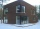Bob traveled 20 meters to Dave's house. He arrived in 3 minutes. What was his average speed for the trip.
17. Truck and car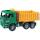At 6h45min the truck started at a speed of 36 km/h. At 7h30m behind it a passenger car. Find the average speed of passenger car if it will catch truck 72 km away from the factory.